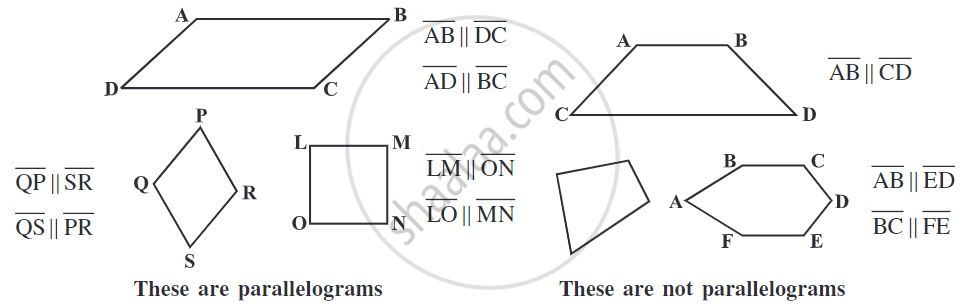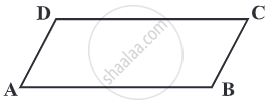# Types of Quadrilaterals - Properties of a Parallelogram

#### definition

Parallelogram: A parallelogram is a quadrilateral whose opposite sides are parallel.

# Parallelogram:

A parallelogram is a quadrilateral whose opposite sides are parallel.

## Properties of a Parallelogram:• A parallelogram has 4 sides.

• The opposite sides of a parallelogram are equal in length.

• The opposite angles of a parallelogram are equal.

• A parallelogram has two diagonals. The diagonals of a parallelogram bisect each other.

• If a pair of opposite sides of a quadrilateral are equal and parallel, it is a parallelogram.

## Element of a Parallelogram:

There are four sides and four angles in a parallelogram.Given a parallelogram ABCD

• Pair of opposite sides:
i) bar(AB) and bar(DC)
ii) bar(AD) and bar(BC)

• Pair of opposite angles:
i) ∠ A and ∠C
ii) ∠ B and ∠ D.

i) bar(AB) and bar(BC)
ii) bar(BC) and bar(CD)
iii) bar(CD) and bar(AD)
iv) bar(AD) and bar(AB) etc.

i) ∠A and ∠B
ii) ∠ B and ∠C
iii) ∠C and ∠D
iv) ∠D and ∠A etc.

If you would like to contribute notes or other learning material, please submit them using the button below.

### Shaalaa.com

What is a Parallelogram? [00:07:19]
S
0%